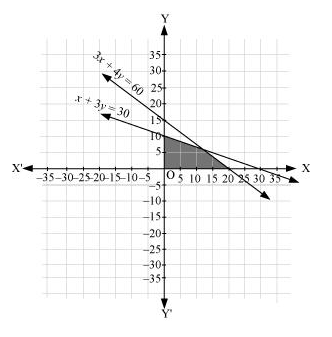# Solve the following system of inequalities graphically: 3x + 4y ≤ 60, x + 3y ≤ 30, x ≥ 0, y ≥ 0

Question:

Solve the following system of inequalities graphically: 3x + 4y  60, x + 3y  30, x  0, y  0

Solution:

$3 x+4 y \leq 60 \ldots(1)$

$x+3 y \leq 30 \ldots(2)$

The graph of the lines, 3x + 4y = 60 and x + 3y = 30, are drawn in the figure below.

Inequality (1) represents the region below the line, 3x + 4y = 60 (including the line 3x + 4y = 60), and inequality (2) represents the region below the line, x + 3y = 30 (including the line x + 3y = 30).

Since x  0 and y  0, every point in the common shaded region in the first quadrant including the points on the respective line and the axes represents the solution of the given system of linear inequalities.